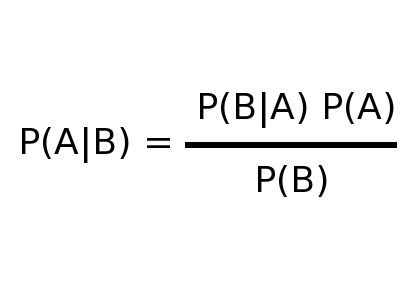# Bayes Theorem

‘In probability theory and applications, Bayes' theorem shows the relation between a conditional probability and its reverse form. For example, the probability of a hypothesis given some observed pieces of evidence and the probability of that evidence given the hypothesis.

The equation used is:Where:

Courtesy of Wikipedia